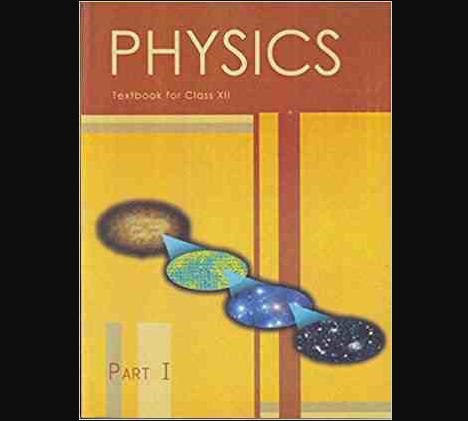# Chapter 8 – Electromagnetic Waves Questions and Answers: NCERT Solutions for Class 12 Physics

Class 12 Physics NCERT book solutions for Chapter 8 - Electromagnetic Waves Questions and Answers.## 9. The Terminology of Different Parts of the Electromagnetic Spectrum Is Given in the Text. Use the Formula E = hv (for Energy of a Quantum of Radiation: Photon) and Obtain the Photon Energy in Units of ev for Different Parts of the Electromagnetic Spectrum. in What Way are the Different Scales of Photon Energies That You Obtain Related to the Sources of Electromagnetic Radiation?

### Ans: The energy of photon can be given as: E=hν=hcλE=hν=hcλ Where, hh = Planck’s constant =6.6×10−34Js=6.6×10−34Js cc = speed of light =3×108m/s=3×108m/s λλ = wavelength of radiation ∴E=6.6×10−34×3×108λJ∴E=6.6×10−34×3×108λJ E=19.8×10−26λJE=19.8×10−26λJ E=19.8×10−26λ×1.6×10−19eVE=19.8×10−26λ×1.6×10−19eV E=12.375×10−7λeVE=12.375×10−7λeV For Different Values of in an Electromagnetic Spectrum, Photon Energies are Listed in Below Table:

 λ(m)λ(m) E(eV)E(eV) 103103 12.375×10−1012.375×10−10 1 12.375×10−712.375×10−7 10−310−3 12.375×10−412.375×10−4 10−610−6 12.375×10−112.375×10−1 10−810−8 12.375×10112.375×101 10−1010−10 12.375×10312.375×103 10−1210−12 12.375×10512.375×105